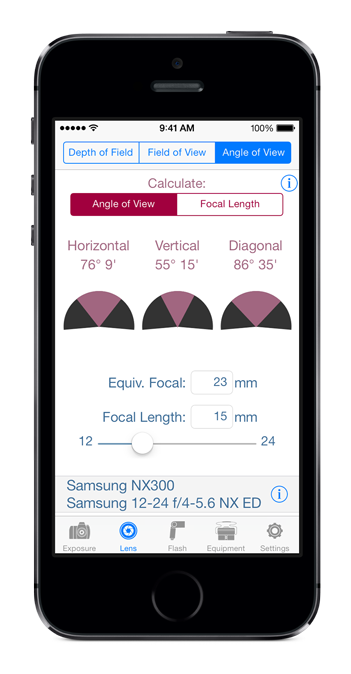Depth of field is the range of distances in a scene that appear acceptably sharp in an image. The level of sharpness acceptability can be tuned by adjusting the Circle of Confusion parameter in the Settings tab.

Near is the nearest point that is acceptably sharp and Far the farthest. DoF field displays the depth of field as the difference between Far and Near.

Hyperfocal distance is the closest focus distance for which the depth of field extends to infinity. Set Hyperfocal button sets the focus distance to hyperfocal distance. The Near value will be approximately half the hyperfocal distance. The Set Hyperfocal button is available only when calculating DoF.

The application can also calculate the Aperture, Focus Distance, and Focal Length for a specific DoF. When the Focus Distance or Focal Length is calculated, the corresponding sliders are disabled.

When DoF is set inf and Calculate is set to Aperture, the app calculates the minimum aperture for which the specified focus distance is bigger than the hyperfocal distance. When Calculate is set to Focal Len. it calculates the maximum focal length for which the hyperfocal distance is smaller than the specified focus distance.

The selected camera and lens are displayed at the bottom of the screen and can be changed by clicking the disclosure button next to them.

When imperial units are selected, you don’t need to use the foot and inch signs. Just enter the feet and inches values separated by a space.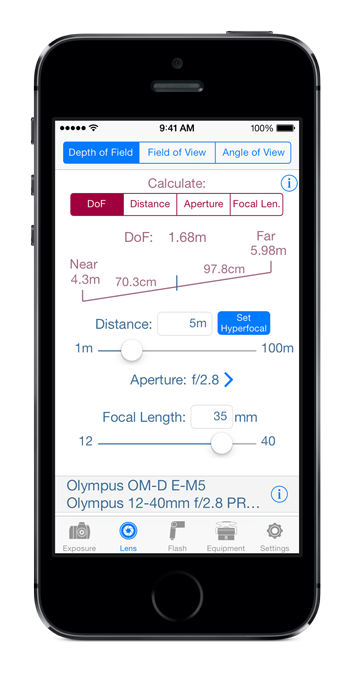Field of view calculates the rectangle size of the scene at a certain distance from the camera that will get recorded on the camera sensor. It also allows finding the combination of camera, lens, and distance that give a certain image rectangle.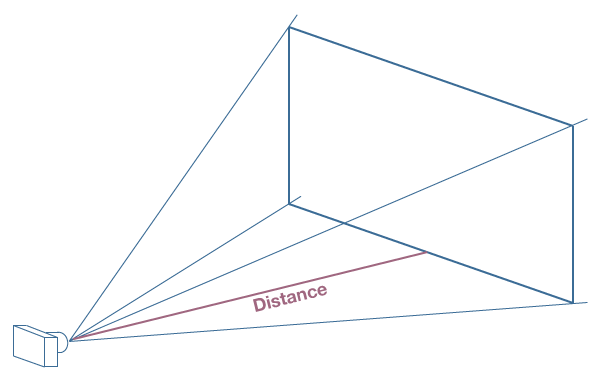For example when using a full frame camera, with an 135mm lens, you need to be 2.5m (8 feet) from the subject to get a vertical image rectangle that is 60cm (2 feet) high, for a head and shoulders portrait.

The image rectangle size is determined by the distance to subject and angle of view, which is determined by the lens focal length, and the camera sensor size.

The application can calculate the field of view, distance, or focal length, using the other two variables as inputs. When distance or focal length is calculated the corresponding sliders are disabled.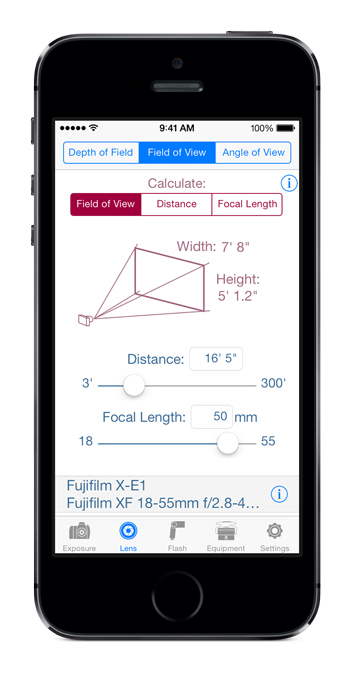Angle of view is the angle over which a scene is recorded by a camera. Angle of view is determined by the lens focal length and the camera sensor size. As a result the angle of view will change when a camera with a different sensor size is selected or when the focal length is changed: the lens is zoomed or a different lens is selected.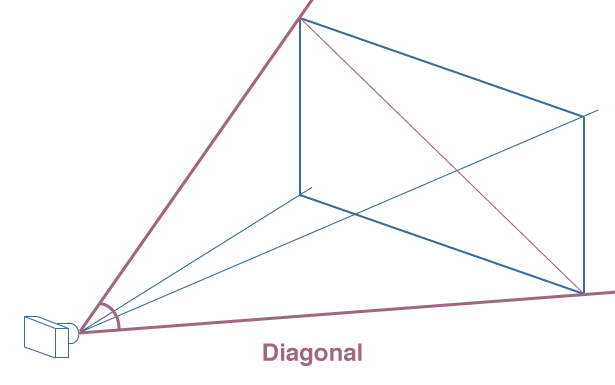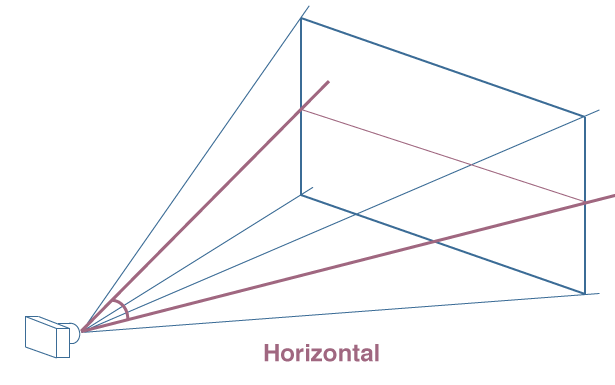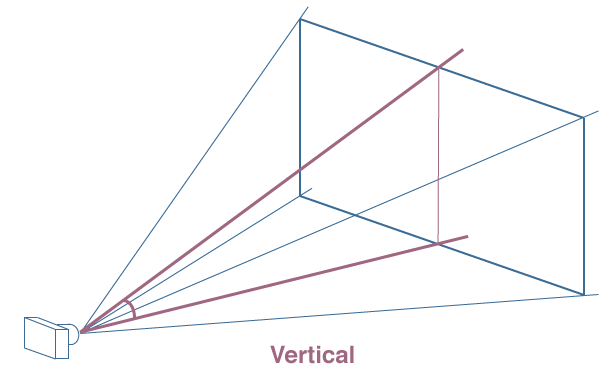Angle of view is directly proportional to sensor size and inversely proportional to focal length. When sensor size is decreased, focal length needs to be decreased to keep the angle of view unchanged. For digital cameras this is called digital multiplier and means that for an APS-C camera one needs to multiply the focal length by 1.5 (1.6 for Canon) to get the equivalent focal length on a full frame camera.

The application can calculate the angle of view for a focal length or the focal length for a specific angle of view. When the focal length is calculated, the focal length slider is disabled.

Equiv. Focal is the 35mm equivalent focal length.

Angle values are displayed and can be entered in decimal degrees or degrees and minutes of arc, depending on the configuration in the Settings tab.

When you enter degree values, you don’t need to use the degree sign. Just enter the degrees and arcminutes values separated by a space without entering the degree or arcminute signs.